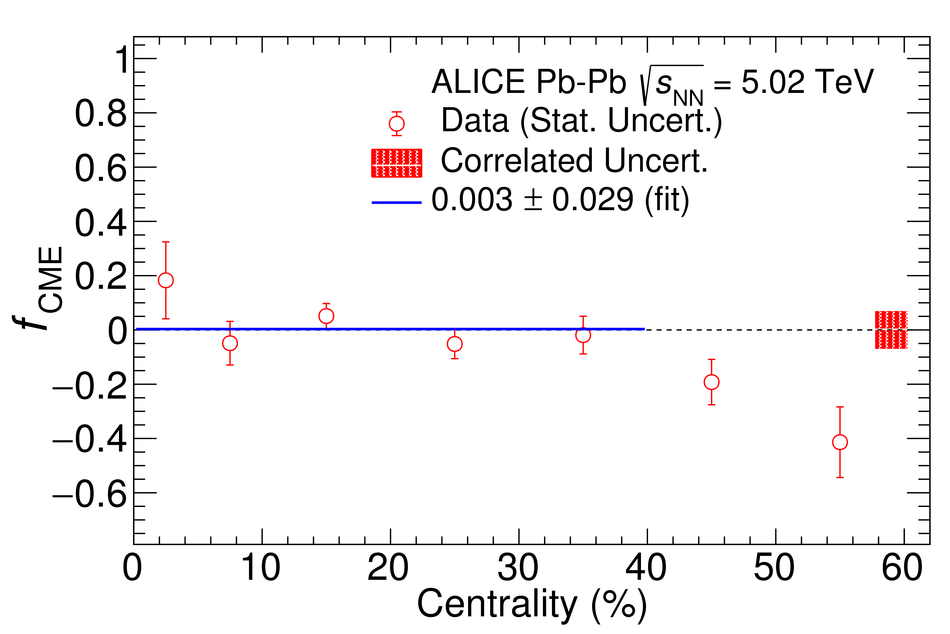# Constraining the Chiral Magnetic Effect with charge-dependent azimuthal correlations in Pb-Pb collisions at $\sqrt{\it{s}_{\mathrm{NN}}}$ = 2.76 and 5.02 TeV

Systematic studies of charge-dependent two- and three-particle correlations in Pb-Pb collisions at $\sqrt{\it{s}_\mathrm{{NN}}} =$ 2.76 and 5.02 TeV used to probe the Chiral Magnetic Effect (CME) are presented. These measurements are performed for charged particles in the pseudorapidity ($\eta$) and transverse momentum ($p_{\rm{T}}$) ranges $\left|\eta \right| <~ 0.8$ and $0.2 <~ p_{\mathrm{T}} <~ 5$ GeV/$c$. A significant charge-dependent signal that becomes more pronounced for peripheral collisions is reported for the CME-sensitive correlators $\gamma_{1,1} = \langle \cos (\varphi_{\alpha} +\varphi_{\beta} - 2\Psi_{2}) \rangle$ and $\gamma_{1,-3} = \langle \cos (\varphi_{\alpha} -3\varphi_{\beta} + 2\Psi_{2}) \rangle$. The results are used to estimate the contribution of background effects, associated with local charge conservation coupled to anisotropic flow modulations, to measurements of the CME. A blast-wave parametrisation that incorporates local charge conservation tuned to reproduce the centrality dependent background effects is not able to fully describe the measured $\gamma_{1,1}$. Finally, the charge and centrality dependence of mixed-harmonics three-particle correlations, of the form $\gamma_{1,2} = \langle \cos (\varphi_{\alpha} +2\varphi_{\beta} - 3\Psi_{3}) \rangle$, which are insensitive to the CME signal, verify again that background contributions dominate the measurement of $\gamma_{1,1}$.

Submitted to: JHEP
e-Print: arXiv:2005.14640 | PDF | inSPIRE
CERN-EP-2020-098

Figures

## Figure 1

 (Left panel): The centrality dependence of $\delta_1$, $\delta_2$, $\delta_3$, and $\delta_4$ for pairs of particles of opposite (OS) and same (SS) sign measured in Pb--Pb collisions at $\sqrt{s_\mathrm{{\rm NN}}} = 5.02$~TeV. (Right panel): The charge-dependent differences, $\Delta \delta_{\mathrm{n}}$ for $n$~=~1,~2,~3~and~4, as a function of collision centrality. The statistical uncertainties for some data points are smaller than the marker size. The systematic uncertainties of each data point are represented by the shaded boxes.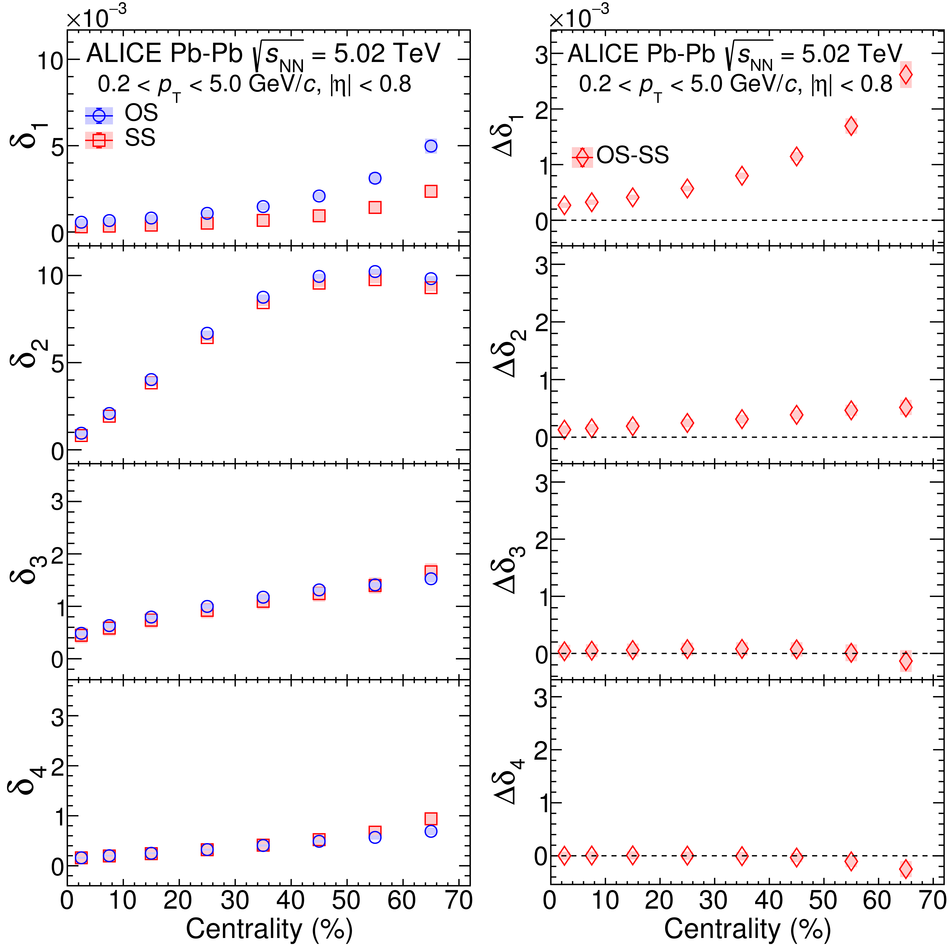## Figure 2

 The dependence of $\delta_1$, $\delta_2$, $\delta_3$ and $\delta_4$ on the transverse momentum difference $\Delta p_{\mathrm{T}} = |p_{\mathrm{T},\alpha} - p_{\mathrm{T},\beta}|$ (left panel), the average transverse momentum $\overline{p}_{\mathrm{T}} = (p_{\mathrm{T},\alpha} + p_{\mathrm{T},\beta})/2$ (middle panel) and the pseudorapidity difference $\Delta\eta = |\eta_{\alpha} - \eta_{\beta}|$ (right panel) of the pair. The results for both opposite (circles) and same sign (squares) particle pairs are reported for one indicative centrality interval (30--40$\%$) of Pb--Pb collisions at $\sqrt{s_\mathrm{{\rm NN}}} = 5.02$~TeV.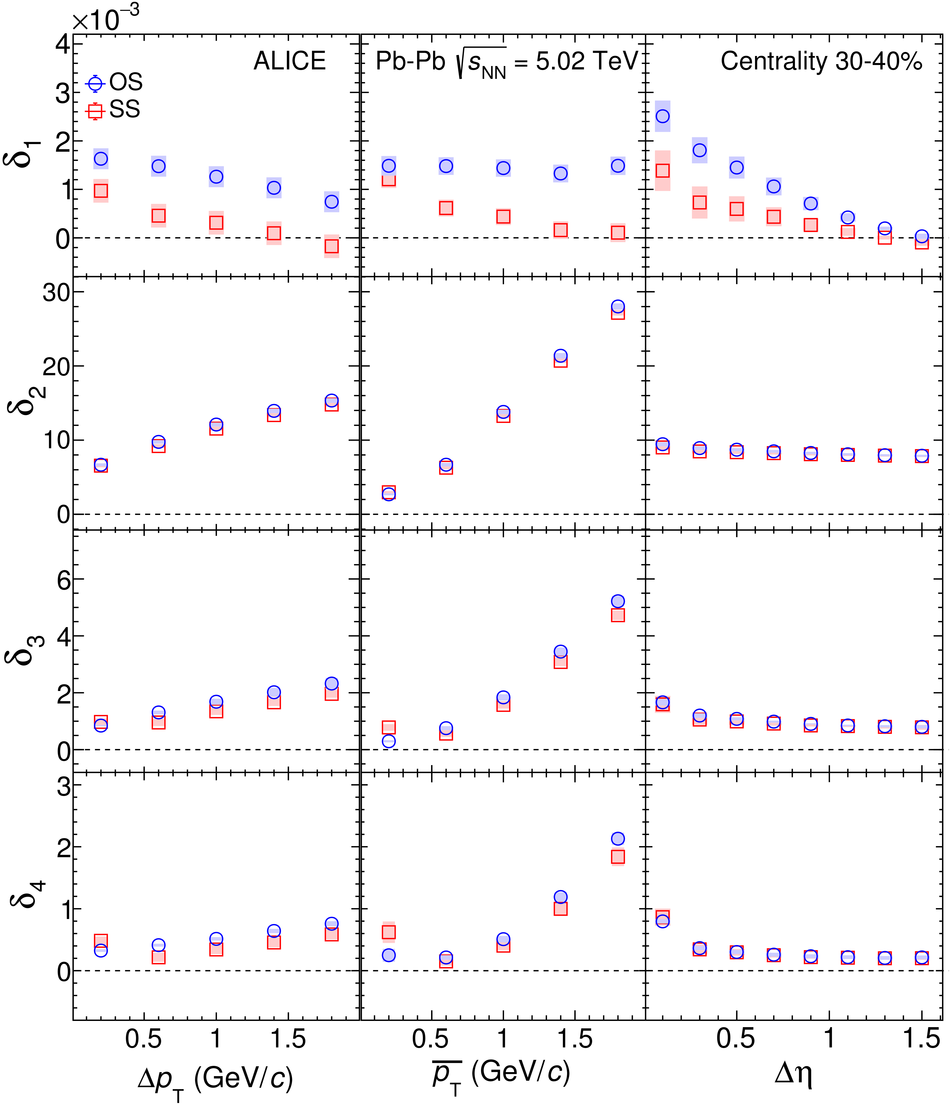## Figure 3

 (Left panel): The centrality dependence of $\gamma_{1,1}$, $\gamma_{1,-3}$, $\gamma_{1,2}$ and $\gamma_{2,2}$ for pairs of particles of opposite (OS) and same (SS) sign measured in Pb--Pb collisions at $\sqrt{s_\mathrm{{\rm NN}}} = 5.02$~TeV. (Right panel): The charge-dependent differences $\Delta \gamma_{1,1}$, $\Delta \gamma_{1,-3}$, $\Delta \gamma_{1,2}$ and $\Delta\gamma_{2,2}$ as a function of collision centrality.## Figure 4

 The dependence of $\gamma_{1,1}$, $\gamma_{1,-3}$, $\gamma_{1,2}$ and $\gamma_{2,2}$ on the transverse momentum difference $\Delta p_{\mathrm{T}} = |p_{\mathrm{T},\alpha} - p_{\mathrm{T},\beta}|$ (left panel), the average transverse momentum $\overline{p}_{\mathrm{T}} = (p_{\mathrm{T},\alpha} + p_{\mathrm{T},\beta})/2$ (middle panel) and the pseudorapidity difference $\Delta\eta = |\eta_{\alpha} - \eta_{\beta}|$ (right panel) of the pair. The results for both opposite and same sign particle pairs are reported for one indicative centrality interval (30--40$\%$) of Pb--Pb collisions at $\sqrt{s_\mathrm{{\rm NN}}} = 5.02$~TeV.## Figure 5

 The dependence of $\Delta \gamma_{1,1}$, $\Delta \gamma_{1,2}$ and $\Delta \gamma_{2,2}$ on centrality, measured in Pb--Pb collisions at $\sqrt{s_\mathrm{{\rm NN}}} = 2.76$~and 5.02~TeV. The data points for $\sqrt{s_\mathrm{{\rm NN}}} = 5.02$~TeV are shifted along the horizontal axis for better visibility.## Figure 6

 (Left) The centrality dependence of $\Delta \delta_1$ measured in Pb--Pb collisions at $\sqrt{s_\mathrm{{\rm NN}}} = 5.02$~TeV. The curve (denoted as BW-LCC) presents the blast-wave parametrization coupled to local charge conservation effects The model is tuned to reproduce the measured values of $\Delta \delta_1$ (see text for details). (Right) The comparison of the centrality dependence of the CME-sensitive correlator $\Delta \gamma_{1,1}$ with expectations from the BW-LCC model.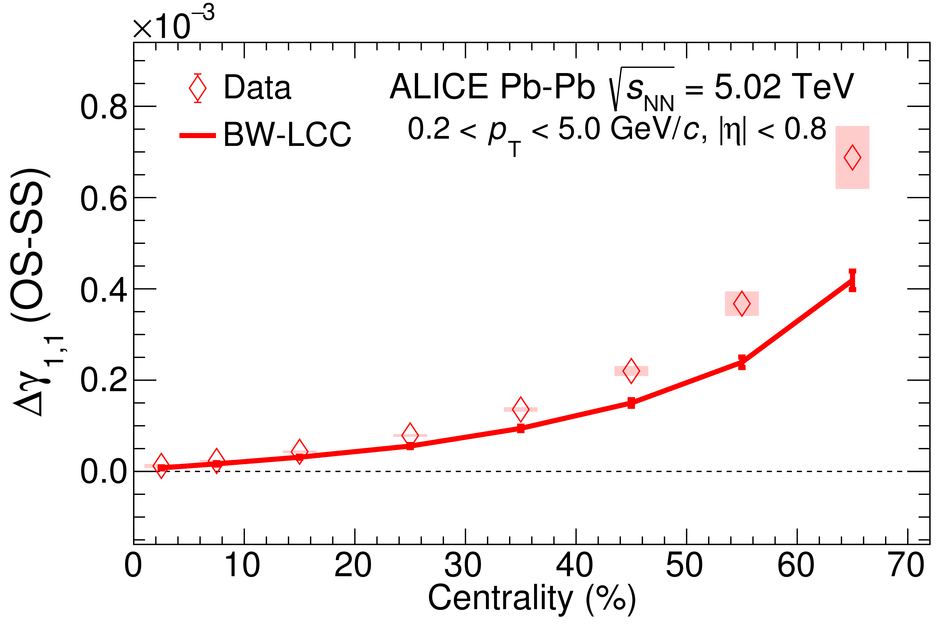## Figure 7

 (Left panel) The centrality dependence of $\Delta\gamma_{1,1}/v_2$ and $\Delta\gamma_{1,2}/v_3$ for Pb--Pb collisions at $\sqrt{s_\mathrm{{\rm NN}}} = 2.76$~TeV according to the AMPT and BW-LCC model. (Right panel) The differences between $\Delta\gamma_{1,1}/v_2$ and $\Delta\gamma_{1,2}/v_3$ in AMPT and BW-LCC, denoted as $\Delta(\Delta\gamma/v_{\mathrm{n}})$ The solid and dotted line is the result of a fit with a constant function to AMPT and BW-LCC result respectively.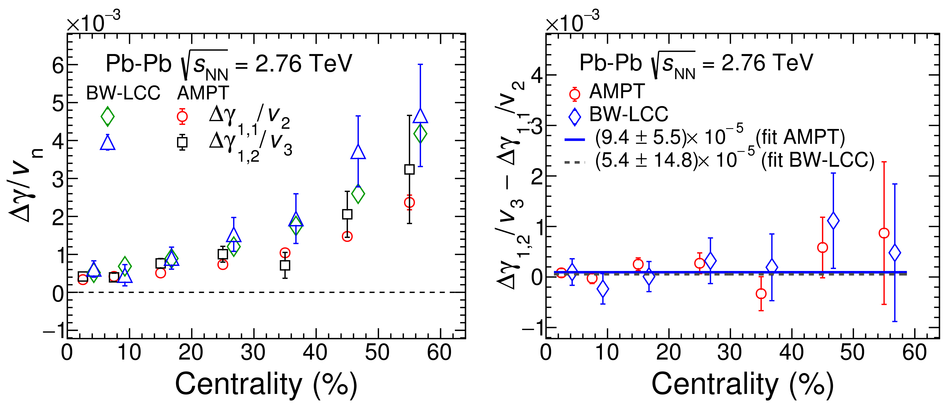## Figure 8

 (Left panel) The CME fraction extracted in Pb--Pb collisions at $\sqrt{s_\mathrm{{\rm NN}}} = 2.76$~TeV. (Right panel) The CME fraction extracted in Pb--Pb collisions at $\sqrt{s_\mathrm{{\rm NN}}} = 5.02$~TeV. The systematic uncertainty is shown as hatched band at zero line around the centrality value of 60\% The solid blue lines correspond to fit with a constant function to the data points. See text for details.